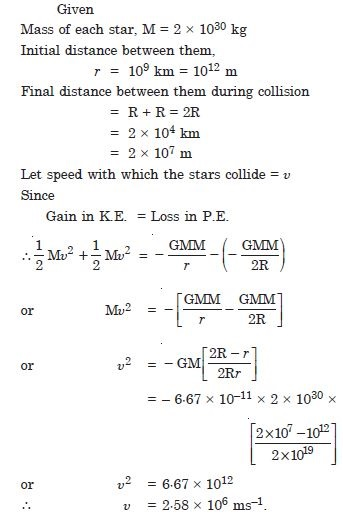# NCERT Solutions for Class 11 Physics Chapter 8 - Gravitation

##### Question 1:

You can shield a charge from electrical forces by putting it inside a hollow conductor. Can you shield a body from the gravitational influence of nearby matter by putting it inside a hollow sphere or by some other means ?

No, because gravitational screens are not possible.

##### Question 2:

An astronaut inside a small spaceship orbiting around the earth cannot detect gravity. If the space station orbiting around the Earth has a large size, can he hope to detect gravity ?

Yes, if the size of the spaceship is large enough for him to detect the variation in g.

##### Question 3:

If you compare the gravitational force on the Earth due to the sun to that due to the moon you would find that the Sun’s pull is greater than the Moon’s pull. However, the tidal effect to the Moon’s pull is greater than the tidal effect of the Sun. Why ?

Tidal effect depends inversely on the cube of the distance unlike gravitational force which depends inversely on the square of the distance.

##### Question 4:

Choose the correct alternative :
Acceleration due to gravity increases/decreases with increasing altitude.

decreases

##### Question 5:

Choose the correct alternative :
Acceleration due to gravity increases/decreases with increasing depth (assume the earth to be a sphere of uniform density).

decreases

##### Question 6:

Choose the correct alternative :
Acceleration due to gravity is independent of the mass of the Earth/mass of the body.

mass of the body

##### Question 7:

Choose the correct alternative :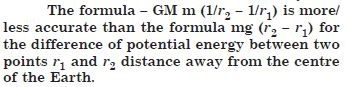more

##### Question 8:

Suppose there existed a planet that went around the sun twice as fast as the earth. What would be its orbital size as compared to that of the earth ?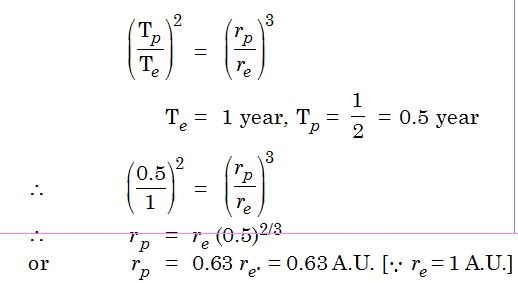##### Question 9:

I0, one of satellites of Jupiter, has an orbital period of 1.769 days and the radius of the orbit is 4·22 × 108 m. Show that mass of the Jupiter is about thousandth that of sun.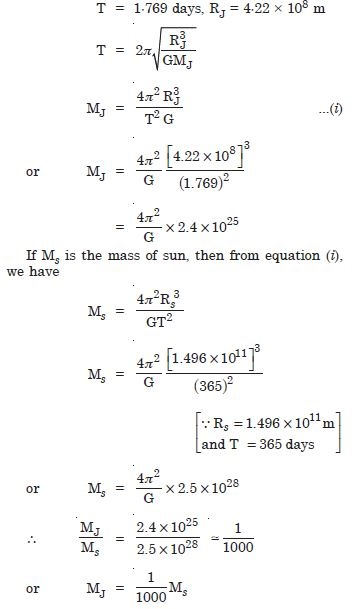##### Question 10:

Let us assume that our galaxy consists of 2.5 × 1011 stars each of one solar mass. How long will a star at a distance of 50000 km from galaxy centre take to complete one revolution ? Take the diameter of the milky way to be 105 ly.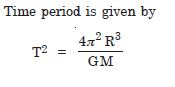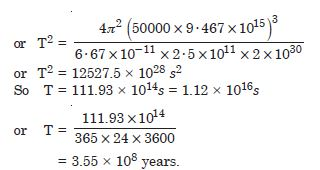##### Question 11:

Choose the correct alternative :
If the zero of potential energy is at infinity the total energy of an orbiting satellite is negative of its kinetic/potential energy.

kinetic energy

##### Question 12:

Choose the correct alternative :
The energy required to rocket an orbiting satellite out of Earth’s gravitational influence is more/less than the energy required to project a stationary object at the same height (as the satellite) out of Earth’s influence.

less

##### Question 13:

Does the escape velocity of a body from the Earth depend on the mass of the body

Since escape velocity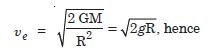The escape velocity is independent of mass of the body.

##### Question 14:

Does the escape velocity of a body from the Earth depend on the location from where it is projected

Since escape velocityNo, it is independent of location from where it is projected.

##### Question 15:

Does the escape velocity of a body from the Earth depend on the direction of projection,

Since escape velocityThe escape velocity is independent of the direction of projection.

##### Question 16:

Does the escape velocity of a body from the Earth depend on the height of the location from where the body is launched ? Explain your answer.

Since escape velocityThe escape velocity depends upon the gravitational potential at the point from where the body is launched. Since this potential depends slightly on the latitude and height of the point, the escape velocity depends slightly on height of location.

##### Question 17:

A comet orbits the sun in a highly elliptical orbit. Does the comet have a constant linear speed

No. The comet does not have a constant linear speed throughout its orbit.

##### Question 18:

A comet orbits the sun in a highly elliptical orbit. Does the comet have a constant angular speed

No. The comet does not have a constant angular speed.

##### Question 19:

A comet orbits the sun in a highly elliptical orbit. Does the comet have a constant angular momentum

Yes. The comet has a constant angular momentum.

##### Question 20:

A comet orbits the sun in a highly elliptical orbit. Does the comet have a constant kinetic energy

No. The comet does not have a constant kinetic energy.

##### Question 21:

A comet orbits the sun in a highly elliptical orbit. Does the comet have a constant potential energy

No, the comet does not have a constant potential energy.

##### Question 22:

A comet orbits the sun in a highly elliptical orbit. Does the comet have a constant total energy throughout its obrit ? Neglect any mass loss of the comet when it comes very close to the Sun.

Yes. The comet has a constant total energy throughout its orbit.

##### Question 23:

Which of the following symptoms is likely to afflict an astronaut in space ?
(a) swollen feet (b) swollen face (c) headache (d) orientational problem.

(b), (c) and (d).

##### Question 24:

The gravitational intensity at the centre of the drum head defined by a hemispherical shell has the direction indicated by the arrow (see figure 8.1) (i) a, (ii) b, (iii) c, (iv) zero.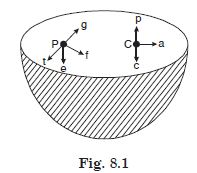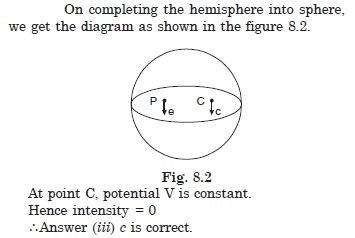##### Question 25:

For the above problem, the direction of the gravitational intensity at any arbitrary point P is indicated by the arrow (i) d, (ii) e, (iii) f, (iv) g.

Since at point P, potential is constant, hence gravitational intensity = 0 $\therefore$ Answer (ii) e is correct.

##### Question 26:

A rocket is fired from the Earth towards the Sun. At what point on its path is the gravitational force on the rocket zero ? Mass of the Sun = 2 × 1030 kg. Mass of the Earth = 6 × 1024 kg. Neglect the effect of other planets etc. (orbital radius = 1·5 × 1011 m).

Let x = distance between the earth and sun
Let P be a point at a distance ‘r’ from the earth where gravitation due to sun and earth is equal and give rise to zero gravitational force.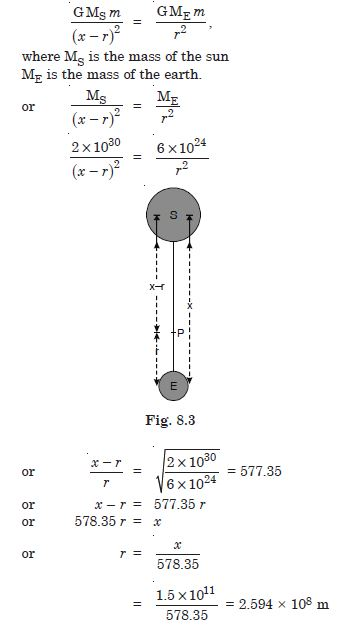##### Question 27:

How will you ‘weigh the Sun’, i.e. estimate its mass ? The mean orbital radius of the Earth around the Sun is 1·5 × 108 km.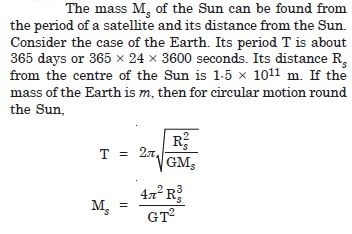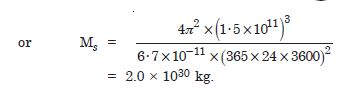##### Question 28:

A saturn year is 29·5 times the earth year. How far is the Saturn from the sun if the earth is 1·50 × 108 km away from the Sun ?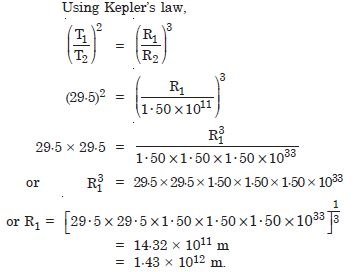##### Question 29:

A body weighs 63 N on the surface of the earth. What is the gravitational force on it at a height equal to half the radius of the earth ?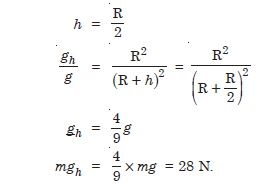##### Question 30:

Assuming the Earth to be a sphere of uniform mass density, how much would a body weigh half way down to the centre of the Earth if it weighed 250 N on the surface ?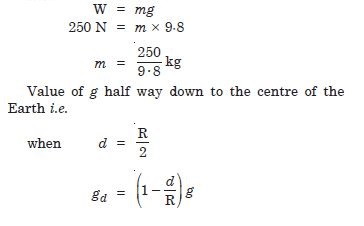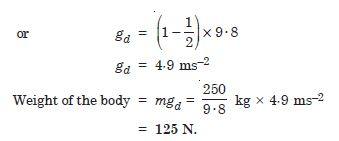##### Question 31: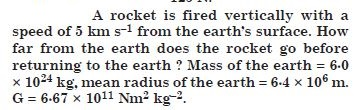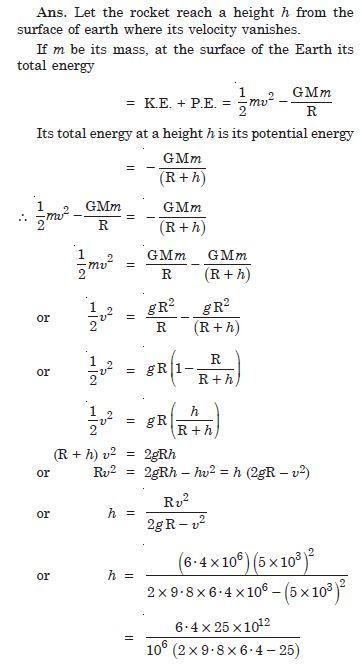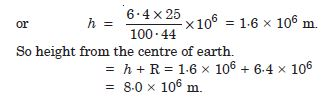##### Question 32:

The escape speed of a projectile on the Earth’s surface is 11·2 km s–1. A body is projected out with thrice this speed. What is the speed of the body far away from the Earth ? Ignore the presence of the Sun and other planets.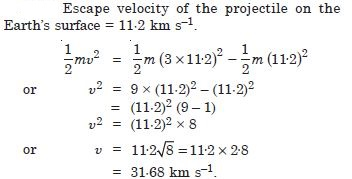##### Question 33: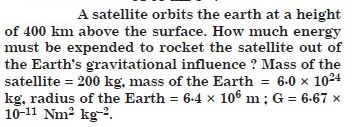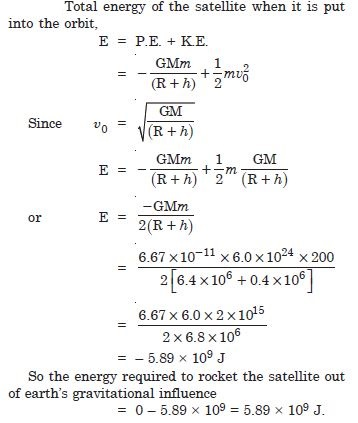##### Question 34:

Two stars each of one solar mass (= 2 × 1030 kg) are approaching each other for a head-on collision. When they are at a distance of 109 km, their speeds are negligible. What is the speed with which they collide ? The radius of each star is 104 km. Assume the stars to remain undistorted until they collide. (Use the known value of G).